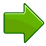# Subtraction

## Subtraction is ...

... taking one number away from another.Start with 5 apples, then subtract 2, we are left with 3 apples.This can be written: 5 − 2 = 3

## Try It Yourself

images/subtract.js

## Train Yourself

You get good at subtraction with practice...

... so use Math Trainer - Subtraction to train yourself!

## Subtraction Table

You can also "look up" answers for simple subtraction using this table:

0 1 2 3 4 5 6 7 8 9 10 11 12
0 0

1 1 0

2 2 1 0

3 3 2 1 0

4 4 3 2 1 0

5 5 4 3 2 1 0

6 6 5 4 3 2 1 0

7 7 6 5 4 3 2 1 0

8 8 7 6 5 4 3 2 1 0

9 9 8 7 6 5 4 3 2 1 0

10 10 9 8 7 6 5 4 3 2 1 0

11 11 10 9 8 7 6 5 4 3 2 1 0
12 12 11 10 9 8 7 6 5 4 3 2 1 0

### Example: Find 8 − 5

• find the row starting with "8"
• move along till you are under the column "5"
• and there is the number "3", so 8 − 5 = 3

## Names

Other names used in subtraction are Minus, Less, Difference, Decrease, Take Away, Deduct.

The names of the numbers in a subtraction fact are:Minuend − Subtrahend = Difference

Minuend: The number that is to be subtracted from.

Subtrahend: The number that is to be subtracted.

Difference: The result of subtracting one number from another.

## Subtracting Larger Numbers

To subtract numbers with more than one digit (such as "42−25") use any of these methods, choose the one you prefer:Subtraction with Regrouping (also called "Borrowing") This is the method most people use!Quick Subtraction (more complicated, but can be faster)Subtraction using Addition (also called the Complements Method)

## Worksheets

For practice try these Subtraction Worksheets

1592, 1593, 3408, 3409, 6619, 6620, 6621, 6622, 6623, 6624, 6625, 6626, 6627, 6628